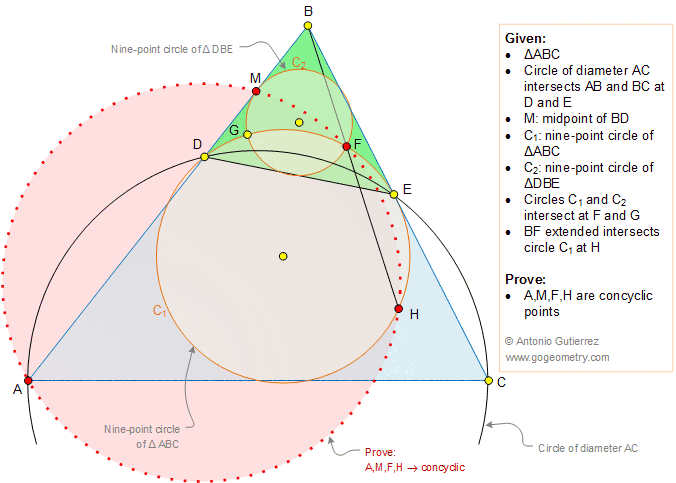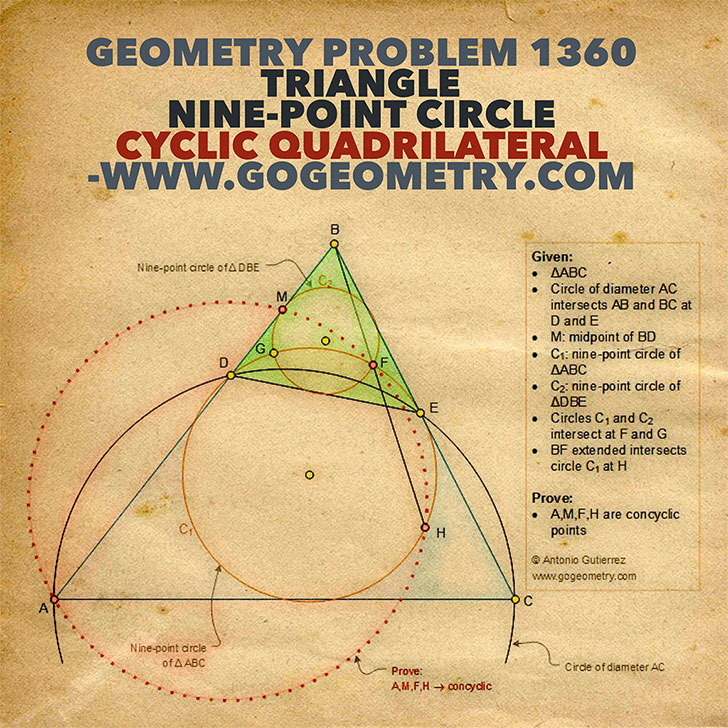# Geometry Problem 1360: Triangle, Nine-Point Circle, Feuerbach's Circle, Euler's Circle, Cyclic Quadrilateral, Concyclic Points, Sketch, iPad Apps.

### Proposition

The figure below shows a triangle ABC. Circle of diameter AC intersects AB and BC at D and E, respectively. C1 is the nine-point circle of triangle ABC and C2 is the nine-point circle of triangle DBE. Circles C1 and C2 intersect at F and G. M is the midpoint of BD and BF extended intersects circle C1 at H. Prove that the points A, M, F, and H are concyclic.### Sketch and Typography of problem 1360 using iPad Apps